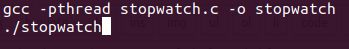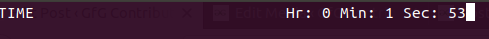# Stopwatch using C language

Create a digital stopwatch program in C which runs on linux base system.

keyboardhit() function simply stands for keyboard hit. After pressing a key it generates a signal and returns a non zero integer. In this, there are 4 loops, 1st loop for hours, 2nd for minutes, 3rd for seconds and 4th loop for maintaining the speed of seconds(3 loop). After running this program it wait for a keyboard start(s) key to be pressed and when key is pressed, it generates a signal. For storing a keyboard key there is a variable(c), if c is equal to p key then it calls the wait function. The thread is running in the background and we are waiting for the start key to be pressed. After pressing s key, the thread again jumps to thread_join function, if r key is pressed, then it jumps into reset label and all the loops are again starts with zeros, if s key is pressed it jumps into start label and if e key is pressed it calls the exit() function and program gets terminated.

Prerequisite : Threads in c, Wait system call

```To execute the program we use following command :```
```Input:
Press a key :
s -> start
e -> exit
r -> reset
p -> pause

Output :```

## Recommended: Please try your approach on {IDE} first, before moving on to the solution.

 `// C code to create stop watch ` ` `  `// Header file for necessary system library ` `#include ` `#include ` `#include ` `#include ` `#include ` `#include ` ` `  `// starting from zero ` `#define MIN 0 ` ` `  `// 1 hr= 60 min ; 1 min= 60 sec ` `#define MAX 60 ` ` `  `#define MILLI 200000 ` ` `  `int` `i, j, k, n, s; ` `char` `c; ` `pthread_t t1; ` ` `  `// Function to perform operations ` `// according keyboeard hit. ` `int` `keyboardhit(``void``) ` `{ ` `    ``struct` `termios oldt, newt; ` `    ``int` `ch; ` `    ``int` `oldf; ` ` `  `    ``tcgetattr(STDIN_FILENO, &oldt); ` `    ``newt = oldt; ` `    ``newt.c_lflag &= ~(ICANON | ECHO); ` `    ``tcsetattr(STDIN_FILENO, TCSANOW, &newt); ` `    ``oldf = fcntl(STDIN_FILENO, F_GETFL, 0); ` `    ``fcntl(STDIN_FILENO, F_SETFL, oldf | O_NONBLOCK); ` ` `  `    ``ch = ``getchar``(); ` ` `  `    ``tcsetattr(STDIN_FILENO, TCSANOW, &oldt); ` `    ``fcntl(STDIN_FILENO, F_SETFL, oldf); ` ` `  `    ``if` `(ch != EOF) { ` `        ``ungetc``(ch, stdin); ` `        ``return` `1; ` `    ``} ` ` `  `    ``return` `0; ` `} ` ` `  `// display stopwatch on screen ` `void` `print() ` `{ ` `    ``// clear screen escape sequence ` `    ``printf``(``"\033[2J\033[1;1H"``); ` ` `  `    ``// Display Hour Min Sec in screen ` `    ``printf``(``"TIME\t\t\t\tHr: %d Min: %d Sec: %d"``, n, i, j); ` `} ` ` `  `// function to pause stopwatch ` `void``* wait(``void``* arg) ` `{ ` ` `  `    ``while` `(1) { ` ` `  `        ``// wait for keyboard signal if keyboard ` `        ``// hit it return non integer number ` `        ``if` `(keyboardhit()) { ` `            ``// take input from user and do ` `            ``// operation accordingly ` `            ``c = ``getchar``(); ` `            ``if` `(c == ``'S'` `|| c == ``'s'``) { ` `                ``break``; ` `            ``} ` `            ``else` `if` `(c == ``'r'` `|| c == ``'R'``) { ` `                ``s = 1; ` `                ``break``; ` `            ``} ` `            ``else` `if` `(c == ``'e'` `|| c == ``'E'``) ` ` `  `                ``exit``(0); ` `        ``} ` `    ``} ` `} ` ` `  `// driver code ` `int` `main() ` `{ ` ` `  `    ``// label to reset the value ` `reset: ` `    ``n = MIN; ` `    ``i = MIN; ` `    ``j = MIN; ` `    ``k = MIN, s = MIN; ` ` `  `    ``print(); ` ` `  `    ``while` `(1) { ` ` `  `        ``/* s for start ` `        ``e for exit ` `        ``p for pause ` `        ``r for reset ` `        ``*/` `        ``if` `(keyboardhit()) { ` `            ``c = ``getchar``(); ` ` `  `            ``if` `(c != ``'s'``) ` `                ``continue``; ` ` `  `            ``for` `(n = MIN; n < MAX; n++) { ` `                ``for` `(i = MIN; i < MAX; i++) { ` `                    ``for` `(j = MIN; j < MAX; j++) { ` `                        ``for` `(k = MIN; k < MILLI; k++) { ` `                        ``start: ` `                            ``print(); ` `                            ``if` `(keyboardhit()) { ` `                                ``c = ``getchar``(); ` ` `  `                                ``if` `(c == ``'r'` `|| c == ``'R'``) ` `                                    ``goto` `reset; ` `                                ``else` `if` `(c == ``'e'` `|| c == ``'E'``) ` `                                    ``exit``(0); ` `                                ``else` `if` `(c == ``'s'` `|| c == ``'S'``) ` `                                    ``goto` `start; ` `                                ``else` `if` `(c == ``'P'` `|| c == ``'p'``) { ` ` `  `                                    ``pthread_create(&t1, NULL, &wait, NULL); ` ` `  `                                    ``// waiting for join a thread ` `                                    ``pthread_join(t1, NULL); ` `                                    ``if` `(s == 1) ` `                                        ``goto` `reset; ` `                                ``} ` `                            ``} ` `                        ``} ` `                    ``} ` `                ``} ` `            ``} ` `        ``} ` `    ``} ` ` `  `    ``return` `0; ` `} `

Output:

``````

My Personal Notes arrow_drop_upCheck out this Author's contributed articles.

If you like GeeksforGeeks and would like to contribute, you can also write an article using contribute.geeksforgeeks.org or mail your article to contribute@geeksforgeeks.org. See your article appearing on the GeeksforGeeks main page and help other Geeks.

Please Improve this article if you find anything incorrect by clicking on the "Improve Article" button below.

Improved By : pixahosod

Article Tags :
Practice Tags :

Be the First to upvote.

Please write to us at contribute@geeksforgeeks.org to report any issue with the above content.